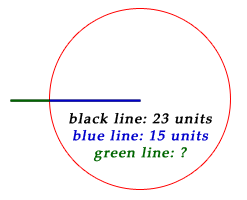SEARCH HOMEMath Central Quandaries & QueriesQuestion from Christine, a parent: I need the inequality to describe the third side of a triange if two sides are known. First side is 23 and second side is 15. What is the third side? Thank you.Hi Christine,

For ease of demonstration, because I'm going to suggest you use a ruler to experiment for yourself, suppose we replace 23 and 15 by 3 and 5.

The third side has to have positive length (greater than zero). How long could it be? Take a piece of paper and a ruler and draw line segments of length 3 and 5 (cms (or inches) that meet as an endpoint. As the angle formed gets larger, the other ends get farther apart. How far could they get? Never quite as far as if the two segments formed a straight line because if the angle is less than 180 degrees, then increasing it moves the ends farther apart. How far apart are they when the angle is 180 degrees?

Since the angle needs to be an interior angle of a triangle, and the sum of angles of a triangle is 180 degrees, it must be less than 180 degrees by at least a little bit.Victoria and StephenMath Central is supported by the University of Regina and The Pacific Institute for the Mathematical Sciences.